Documentation

### This is machine translation

Mouseover text to see original. Click the button below to return to the English version of the page.

# estimate

Perform predictor variable selection for Bayesian linear regression models

To estimate the posterior distribution of a standard Bayesian linear regression model, see `estimate`.

## Syntax

``PosteriorMdl = estimate(PriorMdl,X,y)``
``PosteriorMdl = estimate(PriorMdl,X,y,Name,Value)``
``````[PosteriorMdl,Summary] = estimate(___)``````

## Description

example

````PosteriorMdl = estimate(PriorMdl,X,y)` returns the model that characterizes the joint posterior distributions of β and σ2 of a Bayesian linear regression model. `estimate` also performs predictor variable selection.`PriorMdl` specifies the joint prior distribution of the parameters, the structure of the linear regression model, and the variable selection algorithm. `X` is the predictor data and `y` is the response data. `PriorMdl` and `PosteriorMdl` are not the same object type.To produce `PosteriorMdl`, `estimate` updates the prior distribution with information about the parameters that it obtains from the data.`NaN`s in the data indicate missing values, which `estimate` removes using list-wise deletion.```

example

````PosteriorMdl = estimate(PriorMdl,X,y,Name,Value)` uses additional options specified by one or more name-value pair arguments. For example, `'Lambda',0.5` specifies that the shrinkage parameter value for Bayesian lasso regression is `0.5` for all coefficients except the intercept.If you specify `Beta` or `Sigma2`, then `PosteriorMdl` and `PriorMdl` are equal.```

example

``````[PosteriorMdl,Summary] = estimate(___)``` uses any of the input argument combinations in the previous syntaxes and also returns a table that includes the following for each parameter: posterior estimates, standard errors, 95% credible intervals, and posterior probability that the parameter is greater than 0.```

## Examples

collapse all

Consider the multiple linear regression model that predicts US real gross national product (`GNPR`) using a linear combination of industrial production index (`IPI`), total employment (`E`), and real wages (`WR`).

`${\text{GNPR}}_{t}={\beta }_{0}+{\beta }_{1}{\text{IPI}}_{t}+{\beta }_{2}{\text{E}}_{t}+{\beta }_{3}{\text{WR}}_{t}+{\epsilon }_{t}.$`

For all $t$, ${\epsilon }_{t}$ is a series of independent Gaussian disturbances with a mean of 0 and variance ${\sigma }^{2}$.

Assume the prior distributions are:

• For k = 0,...,3, ${\beta }_{k}|{\sigma }^{2}$ has a Laplace distribution with a mean of 0 and a scale of ${\sigma }^{2}/\lambda$, where $\lambda$ is the shrinkage parameter. The coefficients are conditionally independent.

• ${\sigma }^{2}\sim IG\left(A,B\right)$. $A$ and $B$ are the shape and scale, respectively, of an inverse gamma distribution.

Create a prior model for Bayesian lasso regression. Specify the number of predictors, the prior model type, and variable names. Specify these shrinkages:

• `0.01` for the intercept

• `10` for `IPI` and `WR`

• `1e5` for `E` because it has a scale that is several orders of magnitude larger than the other variables

The order of the shrinkages follows the order of the specified variable names, but the first element is the shrinkage of the intercept.

```p = 3; PriorMdl = bayeslm(p,'ModelType','lasso','Lambda',[0.01; 10; 1e5; 10],... 'VarNames',["IPI" "E" "WR"]);```

`PriorMdl` is a `lassoblm` Bayesian linear regression model object representing the prior distribution of the regression coefficients and disturbance variance.

Load the Nelson-Plosser data set. Create variables for the response and predictor series.

```load Data_NelsonPlosser X = DataTable{:,PriorMdl.VarNames(2:end)}; y = DataTable{:,"GNPR"};```

Perform Bayesian lasso regression by passing the prior model and data to `estimate`, that is, by estimating the posterior distribution of $\beta$ and ${\sigma }^{2}$. Bayesian lasso regression uses Markov chain Monte Carlo (MCMC) to sample from the posterior. For reproducibility, set a random seed.

```rng(1); PosteriorMdl = estimate(PriorMdl,X,y);```
```Method: lasso MCMC sampling with 10000 draws Number of observations: 62 Number of predictors: 4 | Mean Std CI95 Positive Distribution ------------------------------------------------------------------------- Intercept | -1.3472 6.8160 [-15.169, 11.590] 0.427 Empirical IPI | 4.4755 0.1646 [ 4.157, 4.799] 1.000 Empirical E | 0.0001 0.0002 [-0.000, 0.000] 0.796 Empirical WR | 3.1610 0.3136 [ 2.538, 3.760] 1.000 Empirical Sigma2 | 60.1452 11.1180 [42.319, 85.085] 1.000 Empirical ```

`PosteriorMdl` is an `empiricalblm` model object that stores draws from the posterior distributions of $\beta$ and ${\sigma }^{2}$ given the data. `estimate` displays a summary of the marginal posterior distributions in the MATLAB® command line. Rows of the summary correspond to regression coefficients and the disturbance variance, and columns correspond to characteristics of the posterior distribution. The characteristics include:

• `CI95`, which contains the 95% Bayesian equitailed credible intervals for the parameters. For example, the posterior probability that the regression coefficient of `IPI` is in [4.157, 4.799] is 0.95.

• `Positive`, which contains the posterior probability that the parameter is greater than 0. For example, the probability that the intercept is greater than 0 is `0.427`.

Plot the posterior distributions.

`plot(PosteriorMdl)`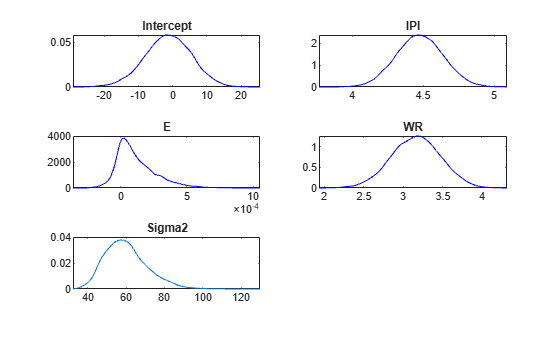Given the shrinkages, the distribution of `E` is fairly dense around 0. Therefore, `E` might not be an important predictor.

By default, `estimate` draws and discards a burn-in sample of size 5000. However, a good practice is to inspect a trace plot of the draws for adequate mixing and lack of transience. Plot a trace plot of the draws for each parameter. You can access the draws that compose the distribution (the properties `BetaDraws` and `Sigma2Draws`) using dot notation.

```figure; for j = 1:(p + 1) subplot(2,2,j); plot(PosteriorMdl.BetaDraws(j,:)); title(sprintf('%s',PosteriorMdl.VarNames{j})); end```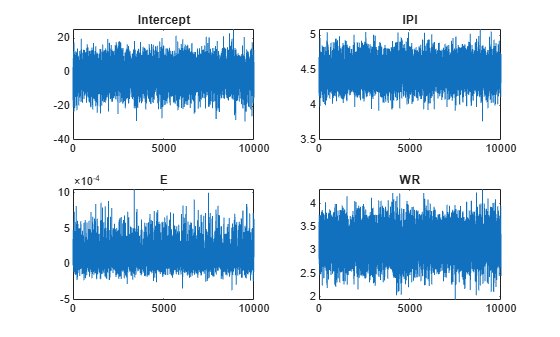```figure; plot(PosteriorMdl.Sigma2Draws); title('Sigma2');```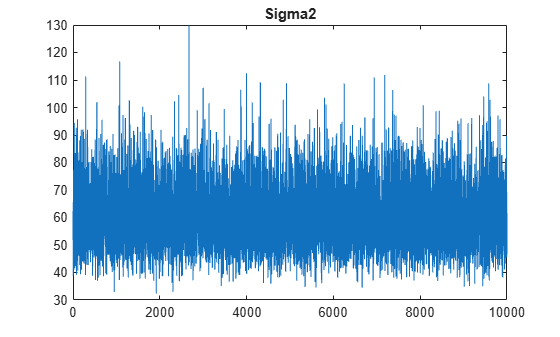The trace plots indicate that the draws seem to mix well. The plots show no detectable transience or serial correlation, and the draws do not jump between states.

Consider the regression model in Select Variables Using Bayesian Lasso Regression.

Create a prior model for performing stochastic search variable selection (SSVS). Assume that $\beta$ and ${\sigma }^{2}\text{\hspace{0.17em}}$are dependent (a conjugate mixture model). Specify the number of predictors `p` and the names of the regression coefficients.

```p = 3; PriorMdl = mixconjugateblm(p,'VarNames',["IPI" "E" "WR"]);```

Load the Nelson-Plosser data set. Create variables for the response and predictor series.

```load Data_NelsonPlosser X = DataTable{:,PriorMdl.VarNames(2:end)}; y = DataTable{:,'GNPR'};```

Implement SSVS by estimating the marginal posterior distributions of $\beta$ and ${\sigma }^{2}$. Because SSVS uses Markov chain Monte Carlo for estimation, set a random number seed to reproduce the results.

```rng(1); PosteriorMdl = estimate(PriorMdl,X,y);```
```Method: MCMC sampling with 10000 draws Number of observations: 62 Number of predictors: 4 | Mean Std CI95 Positive Distribution Regime ---------------------------------------------------------------------------------- Intercept | -18.8333 10.1851 [-36.965, 0.716] 0.037 Empirical 0.8806 IPI | 4.4554 0.1543 [ 4.165, 4.764] 1.000 Empirical 0.4545 E | 0.0010 0.0004 [ 0.000, 0.002] 0.997 Empirical 0.0925 WR | 2.4686 0.3615 [ 1.766, 3.197] 1.000 Empirical 0.1734 Sigma2 | 47.7557 8.6551 [33.858, 66.875] 1.000 Empirical NaN ```

`PosteriorMdl` is an `empiricalblm` model object that stores draws from the posterior distributions of $\beta$ and ${\sigma }^{2}$ given the data. `estimate` displays a summary of the marginal posterior distributions in the command line. Rows of the summary correspond to regression coefficients and the disturbance variance, and columns correspond to characteristics of the posterior distribution. The characteristics include:

• `CI95`, which contains the 95% Bayesian equitailed credible intervals for the parameters. For example, the posterior probability that the regression coefficient of `E` (standardized) is in [0.000, 0.0.002] is 0.95.

• `Regime`, which contains the marginal posterior probability of variable inclusion ($\gamma =1$ for a variable). For example, the posterior probability `E` that should be included in the model is 0.0925.

Assuming that variables with `Regime` < 0.1 should be removed from the model, the results suggest that you can exclude the unemployment rate from the model.

By default, `estimate` draws and discards a burn-in sample of size 5000. However, a good practice is to inspect a trace plot of the draws for adequate mixing and lack of transience. Plot a trace plot of the draws for each parameter. You can access the draws that compose the distribution (the properties `BetaDraws` and `Sigma2Draws`) using dot notation.

```figure; for j = 1:(p + 1) subplot(2,2,j); plot(PosteriorMdl.BetaDraws(j,:)); title(sprintf('%s',PosteriorMdl.VarNames{j})); end```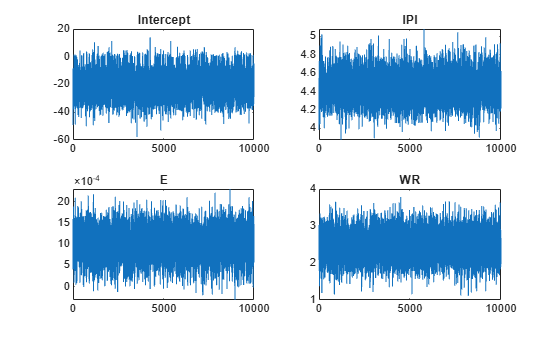```figure; plot(PosteriorMdl.Sigma2Draws); title('Sigma2');```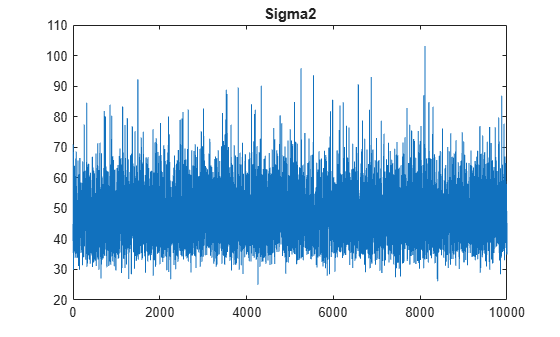The trace plots indicate that the draws seem to mix well. The plots show no detectable transience or serial correlation, and the draws do not jump between states.

Consider the regression model and prior distribution in Select Variables Using Bayesian Lasso Regression.

Create a Bayesian lasso regression prior model for 3 predictors and specify variable names. Specify the shrinkage values `0.01`, `10`, `1e5`, and `10` for the intercept, and the coefficients of `IPI`, `E`, and `WR`.

```p = 3; PriorMdl = bayeslm(p,'ModelType','lasso','VarNames',["IPI" "E" "WR"],... 'Lambda',[0.01; 10; 1e5; 10]);```

Load the Nelson-Plosser data set. Create variables for the response and predictor series.

```load Data_NelsonPlosser X = DataTable{:,PriorMdl.VarNames(2:end)}; y = DataTable{:,"GNPR"};```

Estimate the conditional posterior distributions of $\beta$ given the data and that ${\sigma }^{2}=10$. Return the estimation summary table.

```rng(1); % For reproducibility [Mdl,SummaryBeta] = estimate(PriorMdl,X,y,'Sigma2',10);```
```Method: lasso MCMC sampling with 10000 draws Conditional variable: Sigma2 fixed at 10 Number of observations: 62 Number of predictors: 4 | Mean Std CI95 Positive Distribution ------------------------------------------------------------------------ Intercept | -8.0643 4.1992 [-16.384, 0.018] 0.025 Empirical IPI | 4.4454 0.0679 [ 4.312, 4.578] 1.000 Empirical E | 0.0004 0.0002 [ 0.000, 0.001] 0.999 Empirical WR | 2.9792 0.1672 [ 2.651, 3.305] 1.000 Empirical Sigma2 | 10 0 [10.000, 10.000] 1.000 Empirical ```

`estimate` displays a summary of the conditional posterior distribution of $\beta$. Because ${\sigma }^{2}$ is fixed during estimation, inferences on it are trivial.

Display `Mdl`.

`Mdl`
```Mdl = lassoblm with properties: NumPredictors: 3 Intercept: 1 VarNames: {4x1 cell} Lambda: [4x1 double] A: 3 B: 1 | Mean Std CI95 Positive Distribution --------------------------------------------------------------------------- Intercept | 0 100 [-200.000, 200.000] 0.500 Scale mixture IPI | 0 0.1000 [-0.200, 0.200] 0.500 Scale mixture E | 0 0.0000 [-0.000, 0.000] 0.500 Scale mixture WR | 0 0.1000 [-0.200, 0.200] 0.500 Scale mixture Sigma2 | 0.5000 0.5000 [ 0.138, 1.616] 1.000 IG(3.00, 1) ```

Because `estimate` computes the conditional posterior distribution, it returns the model input `PriorMdl`, not the conditional posterior, in the first position of the output argument list.

Display the estimation summary table.

`SummaryBeta`
```SummaryBeta=5×6 table Mean Std CI95 Positive Distribution Covariances __________ __________ ________________________ ________ ____________ _______________________________________________________________________ Intercept -8.0643 4.1992 -16.384 0.01837 0.0254 'Empirical' 17.633 0.17621 -0.00053724 0.11705 0 IPI 4.4454 0.067949 4.312 4.5783 1 'Empirical' 0.17621 0.0046171 -1.4103e-06 -0.0068855 0 E 0.00039896 0.00015673 9.4925e-05 0.00070697 0.9987 'Empirical' -0.00053724 -1.4103e-06 2.4564e-08 -1.8168e-05 0 WR 2.9792 0.16716 2.6506 3.3046 1 'Empirical' 0.11705 -0.0068855 -1.8168e-05 0.027943 0 Sigma2 10 0 10 10 1 'Empirical' 0 0 0 0 0 ```

`SummaryBeta` contains the conditional posterior estimates.

Estimate the conditional posterior distributions of ${\sigma }^{2}$ given that $\beta$ is the conditional posterior mean of $\beta |{\sigma }^{2},X,y$ (stored in `SummaryBeta.Mean(1:(end – 1))`). Return the estimation summary table.

```condPostMeanBeta = SummaryBeta.Mean(1:(end - 1)); [~,SummarySigma2] = estimate(PriorMdl,X,y,'Beta',condPostMeanBeta);```
```Method: lasso MCMC sampling with 10000 draws Conditional variable: Beta fixed at -8.0643 4.4454 0.00039896 2.9792 Number of observations: 62 Number of predictors: 4 | Mean Std CI95 Positive Distribution ------------------------------------------------------------------------ Intercept | -8.0643 0.0000 [-8.064, -8.064] 0.000 Empirical IPI | 4.4454 0.0000 [ 4.445, 4.445] 1.000 Empirical E | 0.0004 0.0000 [ 0.000, 0.000] 1.000 Empirical WR | 2.9792 0.0000 [ 2.979, 2.979] 1.000 Empirical Sigma2 | 56.8314 10.2921 [39.947, 79.731] 1.000 Empirical ```

`estimate` displays an estimation summary of the conditional posterior distribution of ${\sigma }^{2}$ given the data and that $\beta$ is `condPostMeanBeta`. In the display, inferences on $\beta$ are trivial.

Consider the regression model in Select Variables Using Bayesian Lasso Regression.

Create a prior model for performing SSVS. Assume that $\beta$ and ${\sigma }^{2}\text{\hspace{0.17em}}$are dependent (a conjugate mixture model). Specify the number of predictors `p` and the names of the regression coefficients.

```p = 3; PriorMdl = mixconjugateblm(p,'VarNames',["IPI" "E" "WR"]);```

Load the Nelson-Plosser data set. Create variables for the response and predictor series.

```load Data_NelsonPlosser X = DataTable{:,PriorMdl.VarNames(2:end)}; y = DataTable{:,'GNPR'};```

Implement SSVS by estimating the marginal posterior distributions of $\beta$ and ${\sigma }^{2}$. Because SSVS uses Markov chain Monte Carlo for estimation, set a random number seed to reproduce the results. Suppress the estimation display, but return the estimation summary table.

```rng(1); [PosteriorMdl,Summary] = estimate(PriorMdl,X,y,'Display',false);```

`PosteriorMdl` is an `empiricalblm` model object that stores draws from the posterior distributions of $\beta$ and ${\sigma }^{2}$ given the data. `Summary` is a table with columns corresponding to posterior characteristics and rows corresponding to the coefficients (`PosteriorMdl.VarNames`) and disturbance variance (`Sigma2`).

Display the estimated parameter covariance matrix (`Covariances`) and proportion of times the algorithm includes each predictor (`Regime`).

`Covariances = Summary(:,"Covariances")`
```Covariances=5×1 table Covariances ______________________________________________________________________ Intercept 103.74 1.0486 -0.0031629 0.6791 7.3916 IPI 1.0486 0.023815 -1.3637e-05 -0.030387 0.06611 E -0.0031629 -1.3637e-05 1.3481e-07 -8.8792e-05 -0.00025044 WR 0.6791 -0.030387 -8.8792e-05 0.13066 0.089039 Sigma2 7.3916 0.06611 -0.00025044 0.089039 74.911 ```
`Regime = Summary(:,"Regime")`
```Regime=5×1 table Regime ______ Intercept 0.8806 IPI 0.4545 E 0.0925 WR 0.1734 Sigma2 NaN ```

`Regime` contains the marginal posterior probability of variable inclusion ($\gamma =1$ for a variable). For example, the posterior probability that `E` should be included in the model is 0.0925.

Assuming that variables with `Regime` < 0.1 should be removed from the model, the results suggest that you can exclude the unemployment rate from the model.

## Input Arguments

collapse all

Bayesian linear regression model for predictor variable selection, specified as a model object in this table.

Model ObjectDescription
`mixconjugateblm`Dependent, Gaussian-mixture-inverse-gamma conjugate model for SSVS predictor variable selection, returned by `bayeslm`
`mixsemiconjugateblm`Independent, Gaussian-mixture-inverse-gamma semiconjugate model for SSVS predictor variable selection, returned by `bayeslm`
`lassoblm`Bayesian lasso regression model returned by `bayeslm`

Predictor data for the multiple linear regression model, specified as a `numObservations`-by-`PriorMdl.NumPredictors` numeric matrix. `numObservations` is the number of observations and must be equal to the length of `y`.

Data Types: `double`

Response data for the multiple linear regression model, specified as a numeric vector with `numObservations` elements.

Data Types: `double`

### Name-Value Pair Arguments

Specify optional comma-separated pairs of `Name,Value` arguments. `Name` is the argument name and `Value` is the corresponding value. `Name` must appear inside quotes. You can specify several name and value pair arguments in any order as `Name1,Value1,...,NameN,ValueN`.

Example: `'Sigma2',2` specifies estimating the conditional posterior distribution of the regression coefficients given the data and that the specified disturbance variance is `2`.

Flag to display Bayesian estimator summary to the command line, specified as the comma-separated pair consisting of `'Display'` and a value in this table.

ValueDescription
`true``estimate` prints estimation information and a table summarizing the Bayesian estimators to the command line.
`false``estimate` does not print to the command line.

The estimation information includes the estimation method, fixed parameters, the number of observations, and the number of predictors. The summary table contains estimated posterior means, standard deviations (square root of the posterior variance), 95% equitailed credible intervals, the posterior probability that the parameter is greater than 0, and a description of the posterior distribution (if known). For models that perform SSVS, the display table includes a column for variable-inclusion probabilities.

If you specify either `Beta` or `Sigma2`, then `estimate` includes your specification in the display. Corresponding posterior estimates are trivial.

Example: `'Display',false`

Data Types: `logical`

Value of the regression coefficients for conditional posterior distribution estimation of the disturbance variance, specified as the comma-separated pair consisting of `'Beta'` and a (`PriorMdl.Intercept` + `PriorMdl.NumPredictors`)-by-1 numeric vector. `estimate` estimates the characteristics of π(σ2|`y`,`X`,β = `Beta`), where `y` is `y`, `X` is `X`, and `Beta` is the value of `'Beta'`. If `PriorMdl.Intercept` is `true`, then `Beta(1)` corresponds to the model intercept. All other values correspond to the predictor variables that compose the columns of `X`. `Beta` cannot contain any `NaN` values (that is, all coefficients must be known).

You cannot specify `Beta` and `Sigma2` simultaneously.

By default, `estimate` does not compute characteristics of the conditional posterior of σ2.

Example: `'Beta',1:3`

Data Types: `double`

Value of the disturbance variance for conditional posterior distribution estimation of the regression coefficients, specified as the comma-separated pair consisting of `'Sigma2'` and a positive numeric scalar. `estimate` estimates characteristics of π(β|`y`,`X`,`Sigma2`), where `y` is `y`, `X` is `X`, and `Sigma2` is the value of `'Sigma2'`.

You cannot specify `Sigma2` and `Beta` simultaneously.

By default, `estimate` does not compute characteristics of the conditional posterior of β.

Example: `'Sigma2',1`

Data Types: `double`

Monte Carlo simulation adjusted sample size, specified as the comma-separated pair consisting of `'NumDraws'` and a positive integer. `estimate` actually draws `BurnIn``NumDraws*``Thin` samples. Therefore, `estimate` bases the estimates off `NumDraws` samples. For details on how `estimate` reduces the full Monte Carlo sample, see Algorithms.

Example: `'NumDraws',1e7`

Data Types: `double`

Number of draws to remove from the beginning of the Monte Carlo sample to reduce transient effects, specified as the comma-separated pair consisting of `'BurnIn'` and a nonnegative scalar. For details on how `estimate` reduces the full Monte Carlo sample, see Algorithms.

### Tip

To help you specify the appropriate burn-in period size, determine the extent of the transient behavior in the Monte Carlo sample by specifying `'BurnIn',0`, simulating a few thousand observations using `simulate`, and then plotting the paths.

Example: `'BurnIn',0`

Data Types: `double`

Monte Carlo adjusted sample size multiplier, specified as the comma-separated pair consisting of `'Thin'` and a positive integer.

The actual Monte Carlo sample size is `BurnIn` + `NumDraws``*Thin`. After discarding the burn-in, `estimate` discards every `Thin``1` draws, and then retains the next. For details on how `estimate` reduces the full Monte Carlo sample, see Algorithms.

### Tip

To reduce potential large serial correlation in the Monte Carlo sample, or to reduce the memory consumption of the draws stored in `PosteriorMdl`, specify a large value for `Thin`.

Example: `'Thin',5`

Data Types: `double`

Starting values of the regression coefficients for the Markov chain Monte Carlo (MCMC) sample, specified as the comma-separated pair consisting of `'BetaStart'` and a numeric column vector with (`PriorMdl.Intercept` + `PriorMdl.NumPredictors`) elements. By default, `BetaStart` is the ordinary least-squares (OLS) estimate.

### Tip

A good practice is to run `estimate` multiple times using different parameter starting values. Verify that the solutions from each run converge to similar values.

Example: `'BetaStart',[1; 2; 3]`

Data Types: `double`

Starting values of the disturbance variance for the MCMC sample, specified as the comma-separated pair consisting of `'Sigma2Start'` and a positive numeric scalar. By default, `Sigma2Start` is the OLS residual mean squared error.

### Tip

A good practice is to run `estimate` multiple times using different parameter starting values. Verify that the solutions from each run converge to similar values.

Example: `'Sigma2Start',4`

Data Types: `double`

## Output Arguments

collapse all

Bayesian linear regression model storing distribution characteristics, returned as a `mixconjugateblm`, `mixsemiconjugateblm`, `lassoblm`, or `empiricalblm` model object.

• If you do not specify either `Beta` or `Sigma2` (their values are `[]`), then `estimate` updates the prior model using the data likelihood to form the posterior distribution. `PosteriorMdl` characterizes the posterior distribution and is an `empiricalblm` model object. Information `PosteriorMdl` stores or displays helps you decide whether predictor variables are important.

• If you specify either `Beta` or `Sigma2`, then `PosteriorMdl` equals `PriorMdl` (the two models are the same object storing the same property values). `estimate` does not update the prior model to form the posterior model. However, `Summary` stores conditional posterior estimates.

For more details on the display of `PosteriorMdl`, see `Summary`.

Summary of Bayesian estimators, returned as a table. `Summary` contains the same information as the display of the estimation summary (`Display`). Rows correspond to parameters, and columns correspond to these posterior characteristics:

• `Mean` – Posterior mean

• `Std` – Posterior standard deviation

• `CI95` – 95% equitailed credible interval

• `Positive` – Posterior probability that the parameter is greater than 0

• `Distribution` – Description of the marginal or conditional posterior distribution of the parameter, when known

• `Covariances` – Estimated covariance matrix of the coefficients and disturbance variance

• `Regime` – Variable-inclusion probabilities for models that perform SSVS; low probabilities indicate that the variable should be excluded from the model

Row names are the names in `PriorMdl.VarNames`. The name of the last row is `Sigma2`.

Alternatively, pass `PosteriorMdl` to `summarize` to obtain a summary of Bayesian estimators.

collapse all

### Bayesian Linear Regression Model

A Bayesian linear regression model treats the parameters β and σ2 in the multiple linear regression (MLR) model yt = xtβ + εt as random variables.

For times t = 1,...,T:

• yt is the observed response.

• xt is a 1-by-(p + 1) row vector of observed values of p predictors. To accommodate a model intercept, x1t = 1 for all t.

• β is a (p + 1)-by-1 column vector of regression coefficients corresponding to the variables that compose the columns of xt.

• εt is the random disturbance with a mean of zero and Cov(ε) = σ2IT×T, while ε is a T-by-1 vector containing all disturbances. These assumptions imply that the data likelihood is

`$\ell \left(\beta ,{\sigma }^{2}|y,x\right)=\prod _{t=1}^{T}\varphi \left({y}_{t};{x}_{t}\beta ,{\sigma }^{2}\right).$`

ϕ(yt;xtβ,σ2) is the Gaussian probability density with mean xtβ and variance σ2 evaluated at yt;.

Before considering the data, you impose a joint prior distribution assumption on (β,σ2). In a Bayesian analysis, you update the distribution of the parameters by using information about the parameters obtained from the likelihood of the data. The result is the joint posterior distribution of (β,σ2) or the conditional posterior distributions of the parameters.

## Tips

• Monte Carlo simulation is subject to variation. If `estimate` uses Monte Carlo simulation, then estimates and inferences might vary when you call `estimate` multiple times under seemingly equivalent conditions. To reproduce estimation results, before calling `estimate`, set a random number seed by using `rng`.

## Algorithms

This figure shows how `estimate` reduces the Monte Carlo sample using the values of `NumDraws`, `Thin`, and `BurnIn`.Rectangles represent successive draws from the distribution. `estimate` removes the white rectangles from the Monte Carlo sample. The remaining `NumDraws` black rectangles compose the Monte Carlo sample.